# To check: whether the triangles are similar. If so, write a similarity statement. Given: The given triangles are: 12210203171.jpgQuestion
SimilarityTo check: whether the triangles are similar. If so, write a similarity statement.
Given:
The given triangles are: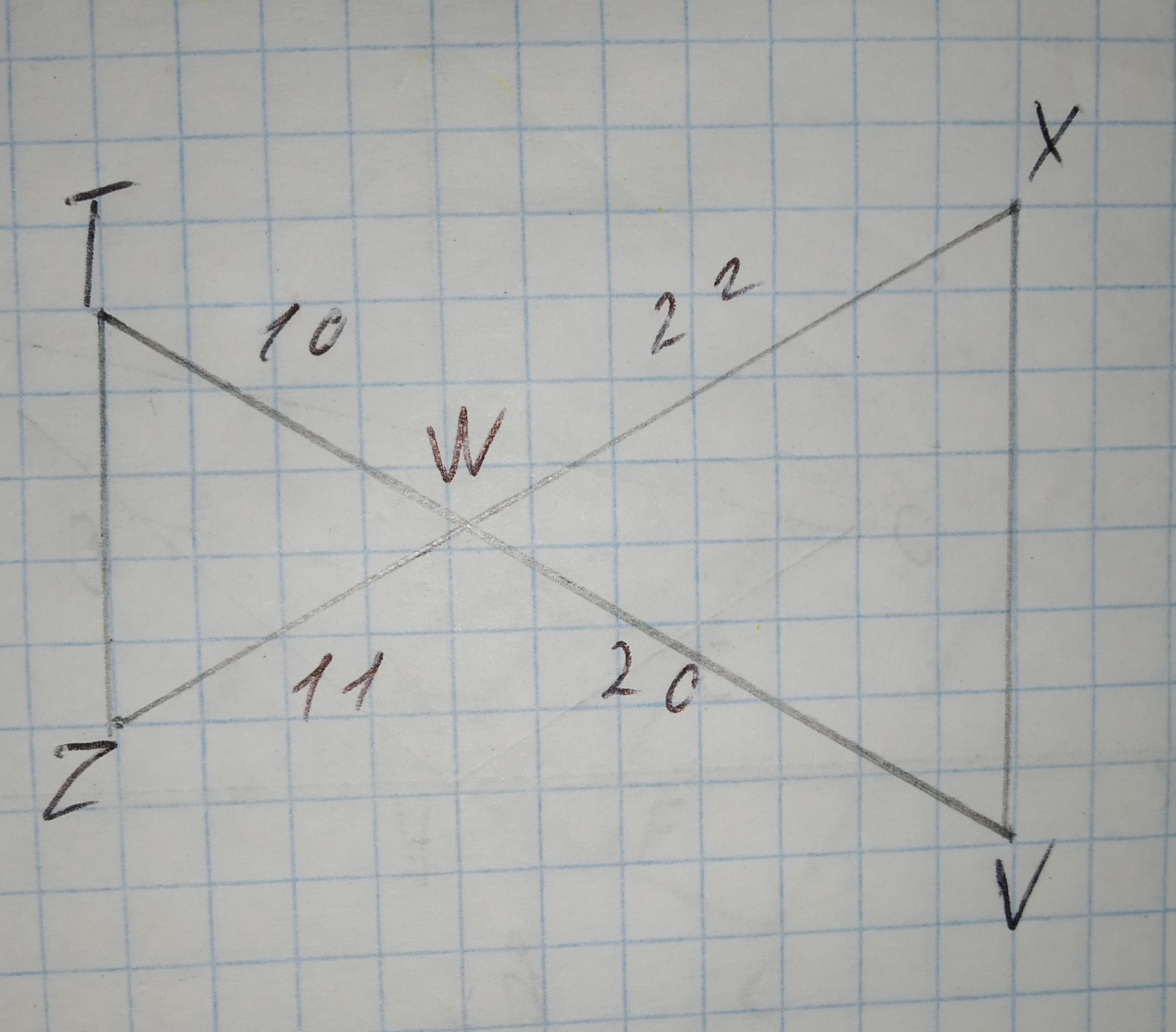2020-10-28
Calculation:
The lenght of the given triangles $$\displaystyle\triangle{T}{W}{Z}$$ are 10,11, and triangles $$\displaystyle\triangle{V}{W}{X}$$ are 20,20. Now,
$$\displaystyle\frac{{{T}{W}}}{{{W}{V}}}=\frac{{10}}{{20}}=\frac{{1}}{{2}}{\left\lbrace{s}{i}{d}{e}{r}{a}{t}{i}{o}\right\rbrace}$$
$$\displaystyle\frac{{{Z}{W}}}{{{X}{W}}}=\frac{{11}}{{22}}=\frac{{1}}{{2}}{\left\lbrace{s}{i}{d}{e}{r}{a}{t}{i}{o}\right\rbrace}$$
$$\displaystyle\angle{T}{W}{Z}=\angle{V}{W}{X}$$
$$\displaystyle\therefore\triangle{T}{W}{Z}\sim\triangle{V}{W}{X}$$ {by SAS-similarity}
Hence, the triangle is similar by SAS-similarity.

### Relevant QuestionsGiven $$\displaystyle\triangle{D}{E}{F}{\quad\text{and}\quad}\triangle{E}{G}{F}$$ in the diagram below, determine if the triangles are similar. If so, write a similarity statement, and state the criterion used to support your claim.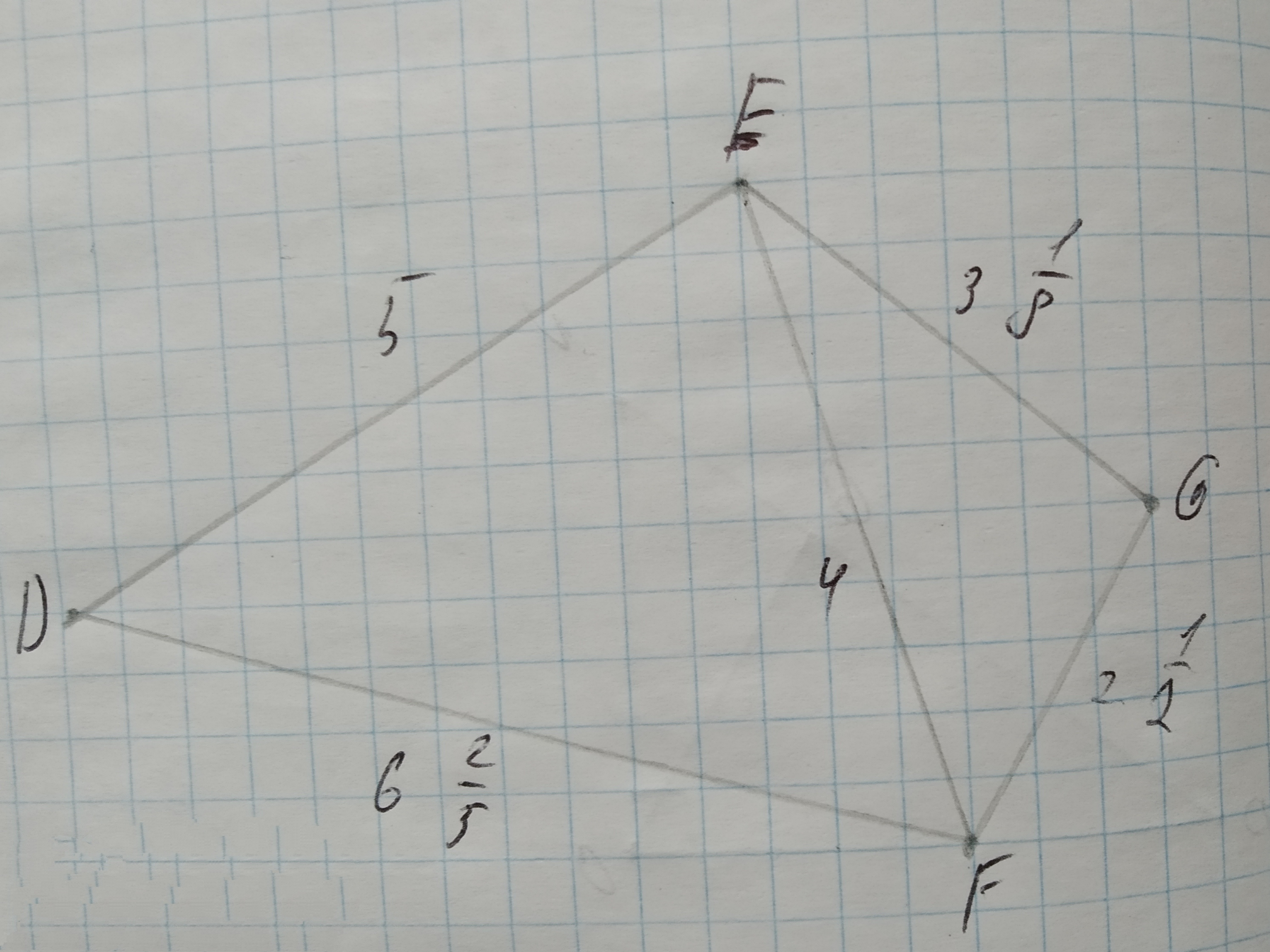Determine whether the triangles are similar.
If so, write a similarity statement and name the postulate or theorem you used. If not, explain.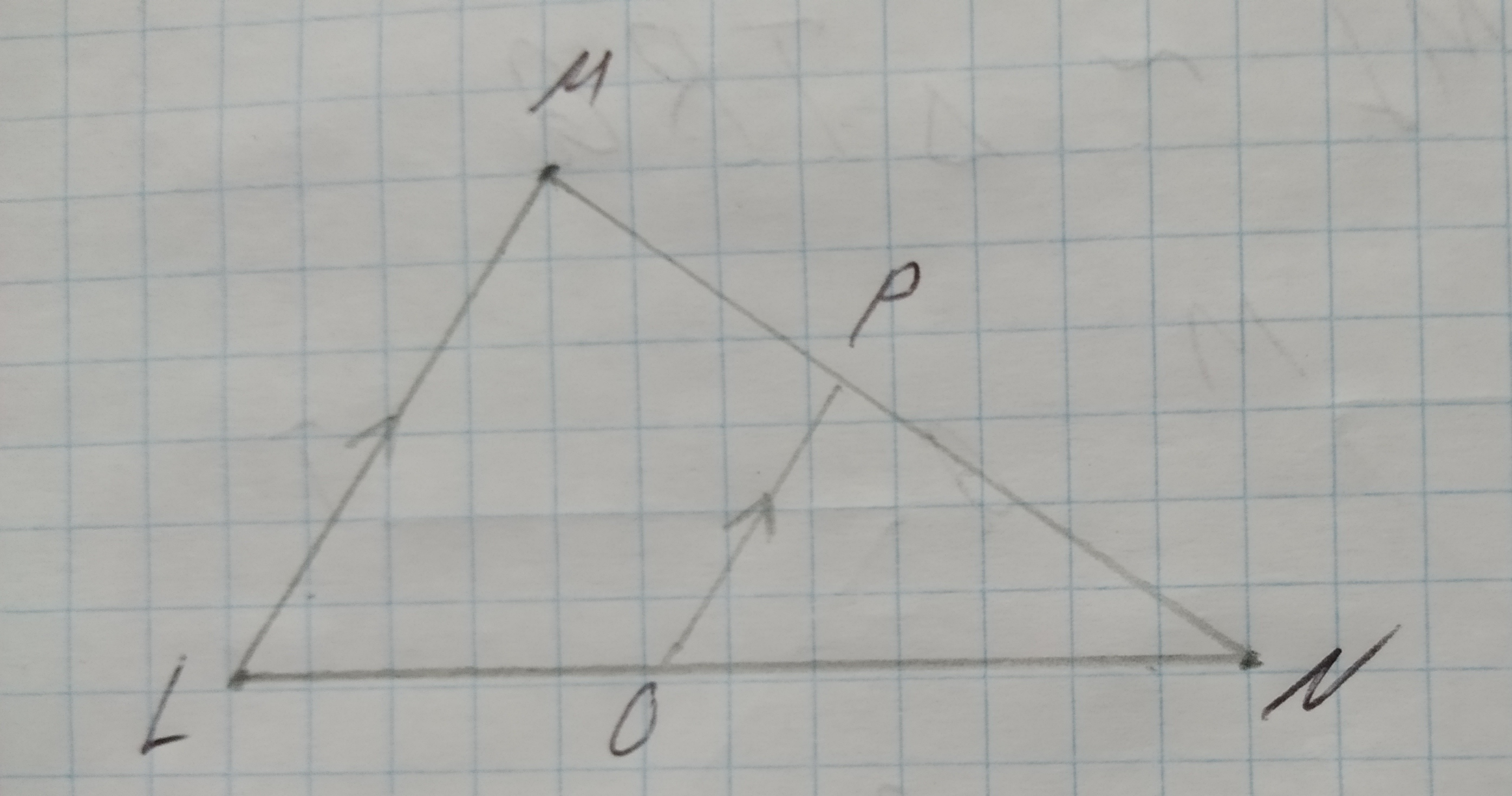Whether the triangle are similar. if so write the similarity statement and if not what would be the sufficient to prove the triangle similar.
Given: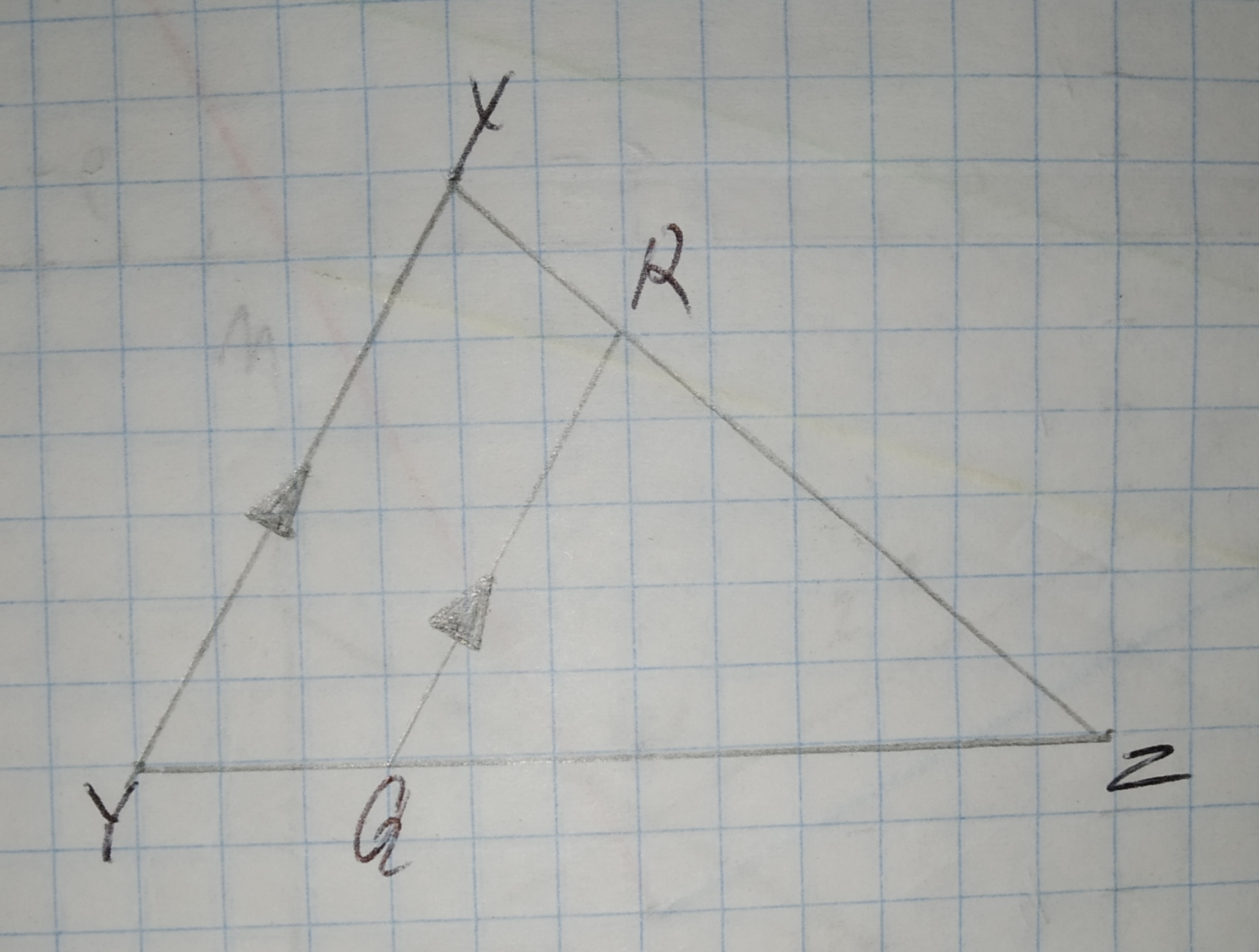To determine:Whether each pair of figures is similar and also write similarity statement and scale factor.
Given information:
Given the figure.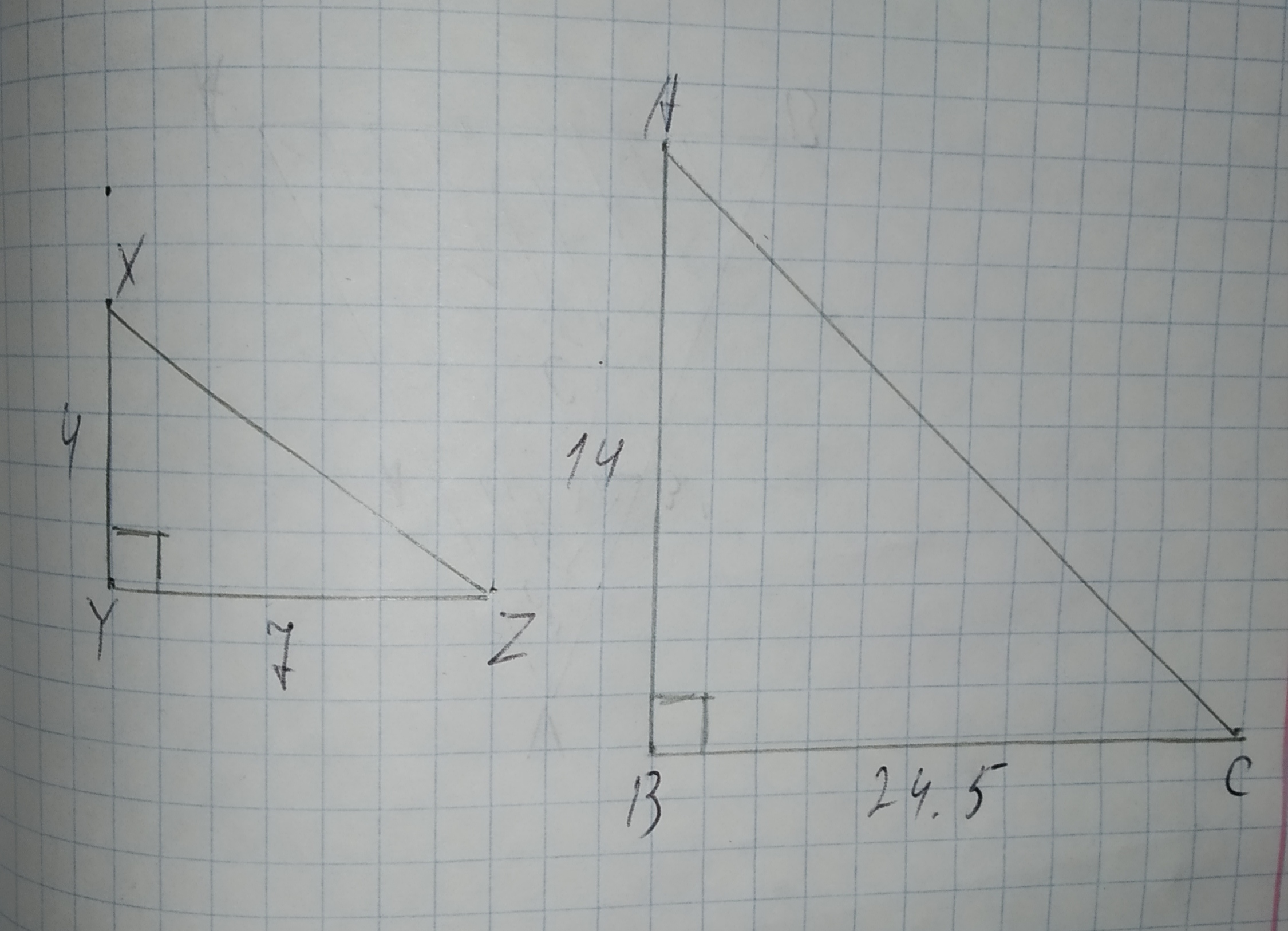To write three similarity statements about the triangles and the reason that they are similar.
Given that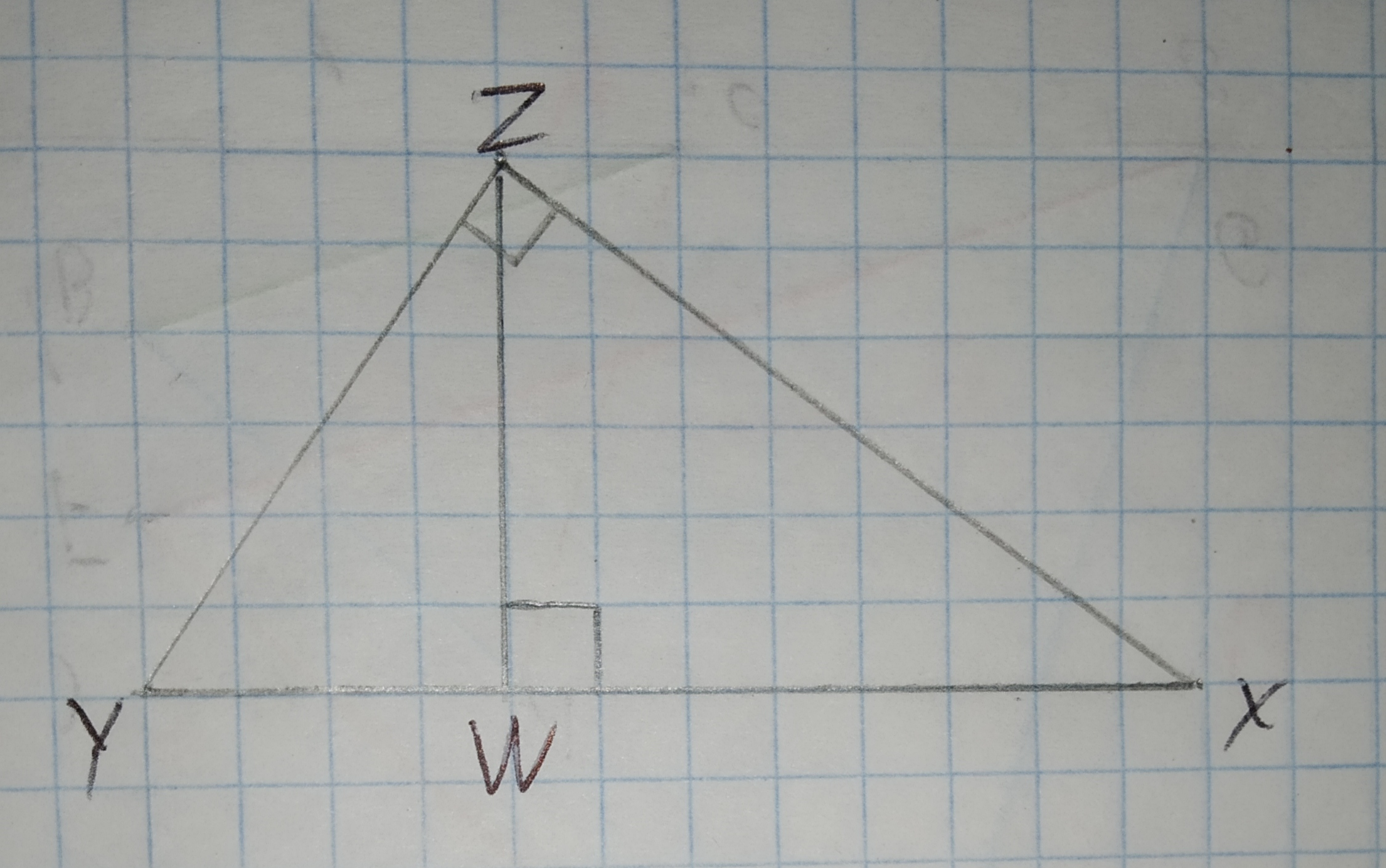To check: whether the additional information in the given option would be enough to prove the given similarity.
Given:
The given similarity is $$\displaystyle\triangle{A}{D}{C}\sim\triangle{B}{E}{C}$$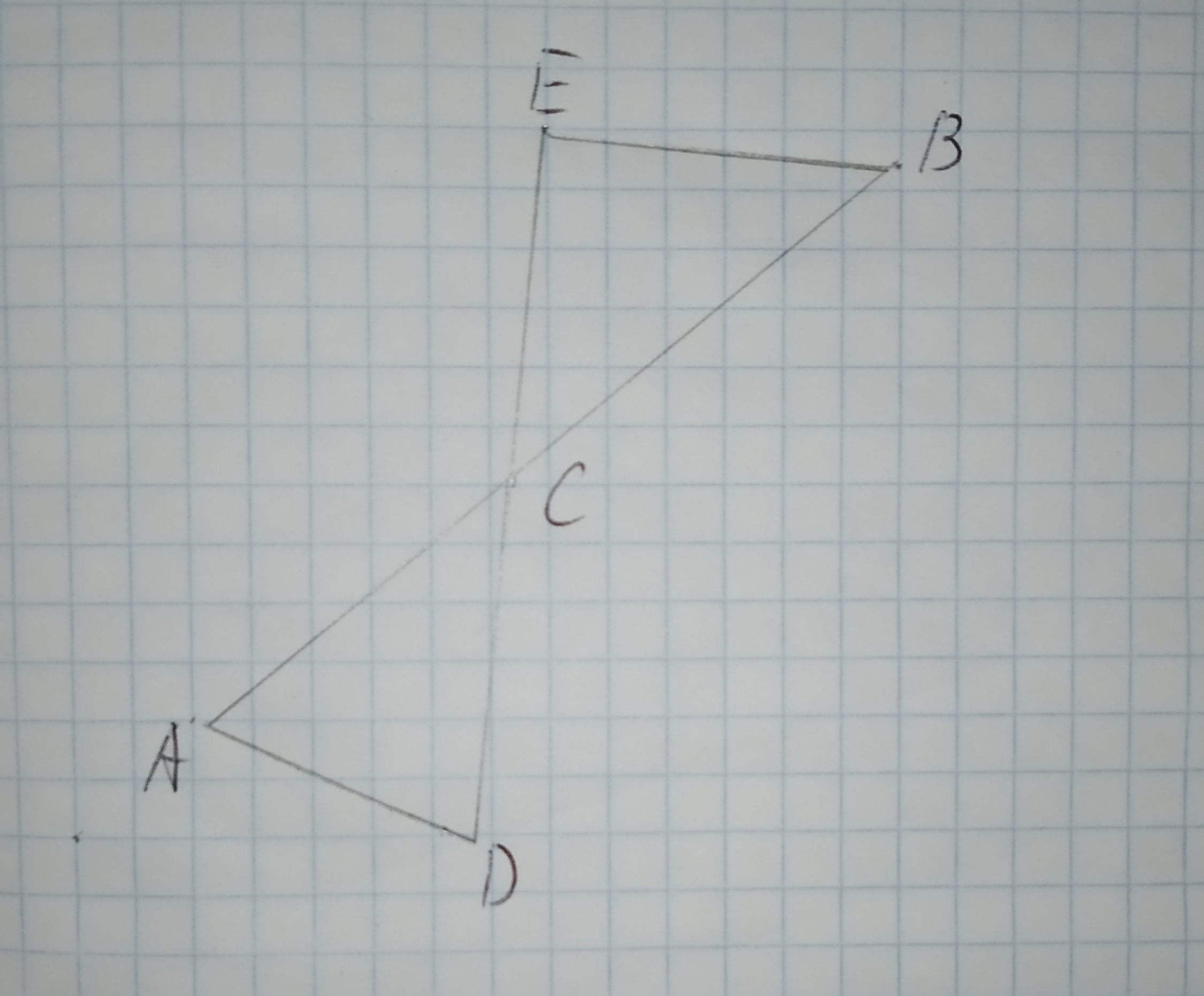The given options are:
A.$$\displaystyle\angle{D}{A}{C}{\quad\text{and}\quad}\angle{E}{C}{B}$$ are congruent.
B.$$\displaystyle\overline{{{A}{C}}}{\quad\text{and}\quad}\overline{{{B}{C}}}$$ are congruent.
C.$$\displaystyle\overline{{{A}{D}}}{\quad\text{and}\quad}\overline{{{E}{B}}}$$ are parallel.
D.$$\displaystyle\angle{C}{E}{B}$$ is a right triangle.Using Similarity Express x in terms of a, b, and c.
Given:
The given pair of triangles is similar.
The given pair of triangle is,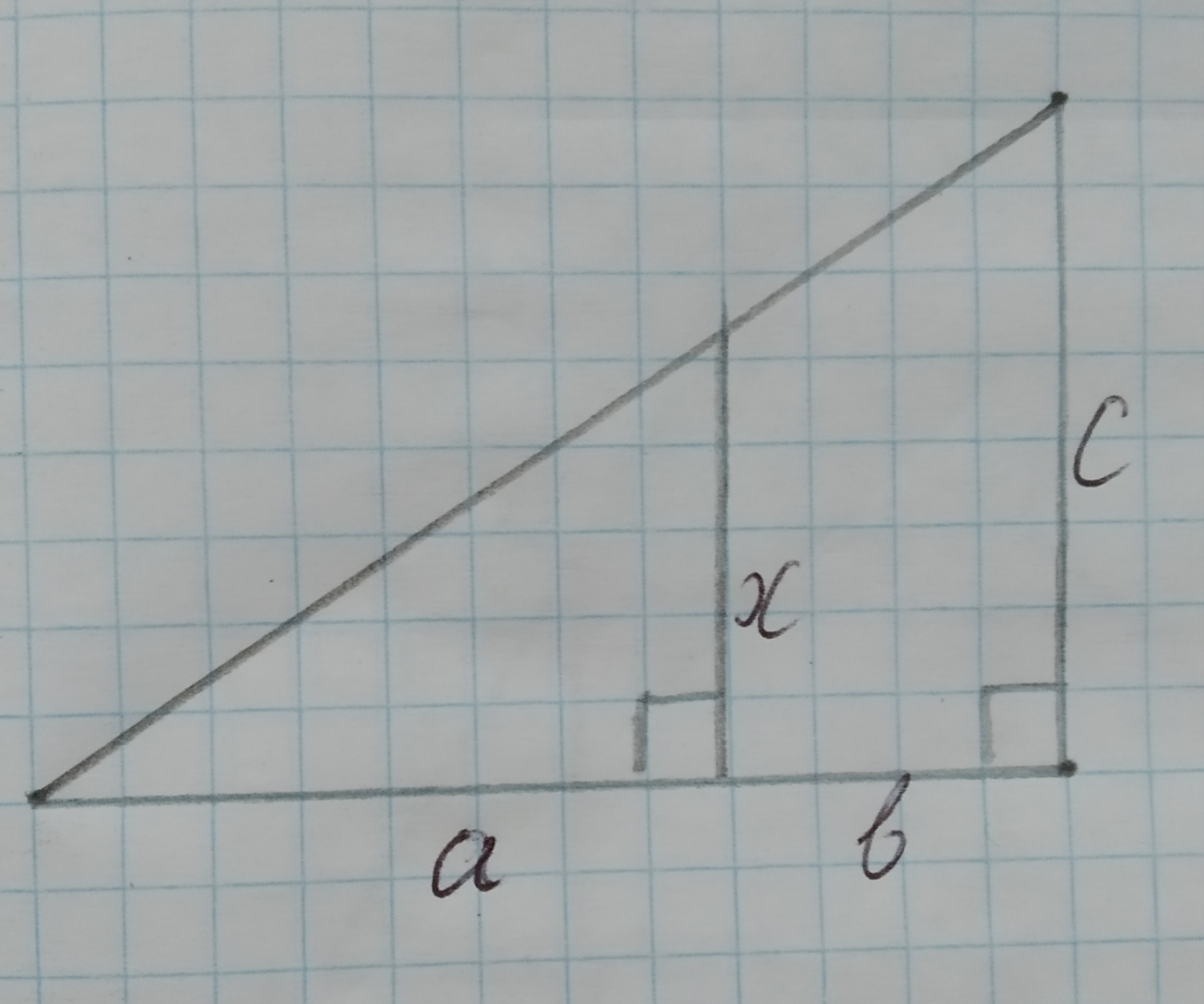Write a similarity statement relating the three triangles in the diagram.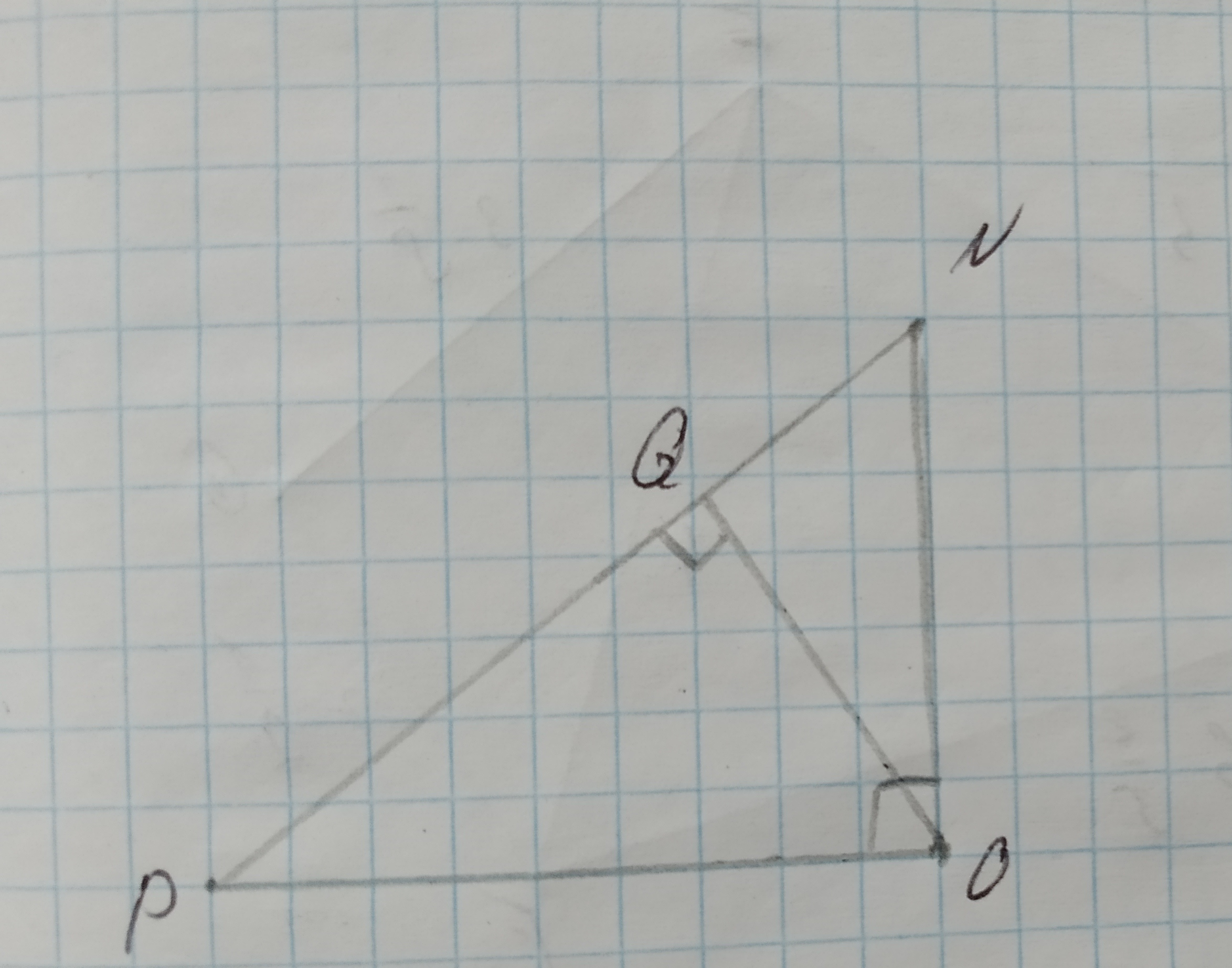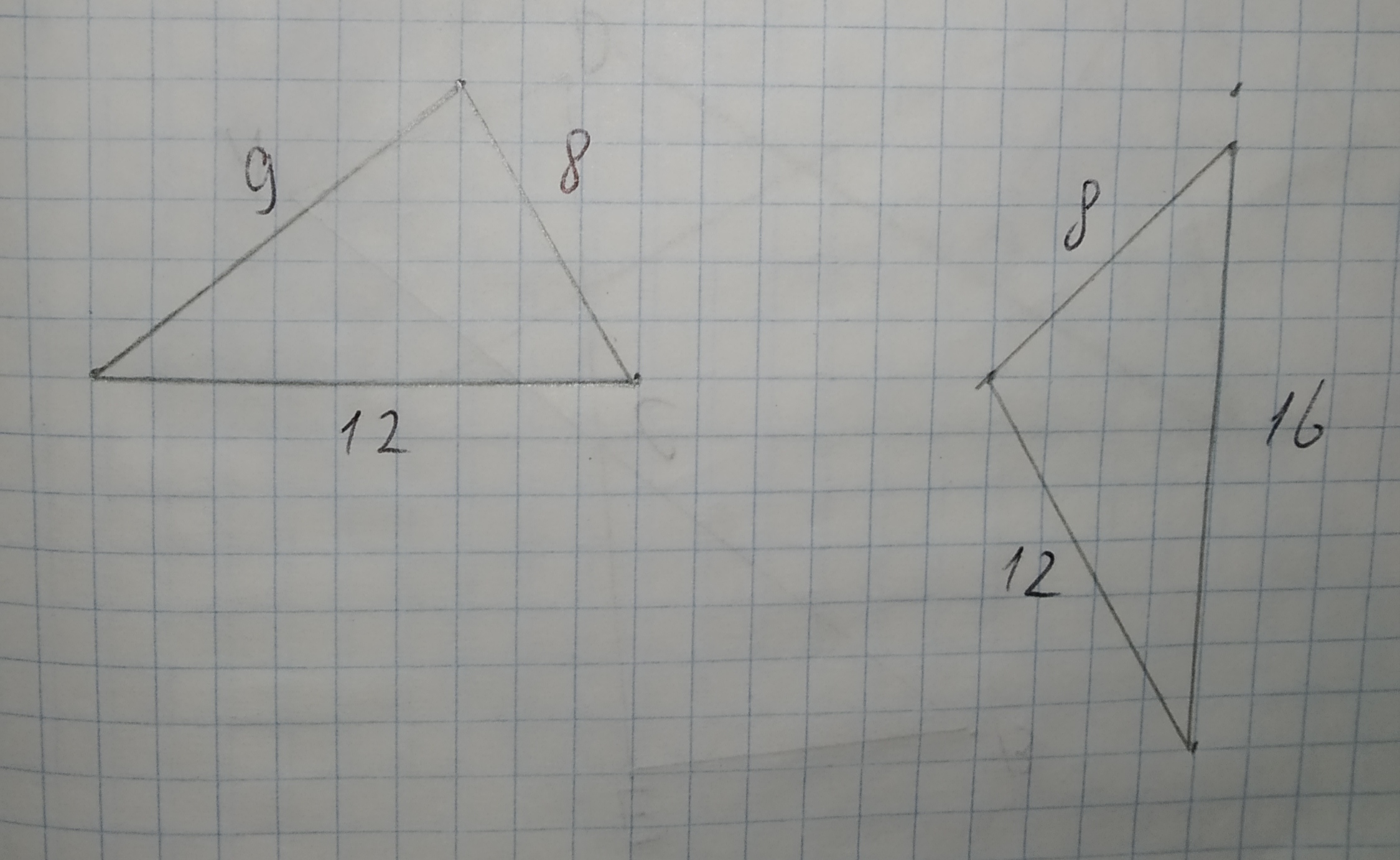Determine whether $$\displaystyle\triangle{A}{B}{C}{\quad\text{and}\quad}\triangle{D}{E}{F}$$ are similar for each set of measures. If so, identify the similarity criterion.
1.$$\displaystyle{m}\angle{A}={20},{m}\angle{C}={20},{m}\angle{D}={40},{m}\angle{F}={40}$$
2.$$\displaystyle{m}\angle{A}={20},{m}\angle{C}={4}{u},{m}\angle{D}={20},{m}\angle{F}={40}$$
3.$$\displaystyle{A}{B}={20},{B}{C}={40},{m}\angle{B}={53}$$,
$$\displaystyle{D}{E}={10},{E}{F}={20},{m}\angle{E}={53}$$